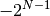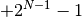# numpy.invert¶

numpy.invert(x[, out])

Compute bit-wise inversion, or bit-wise NOT, element-wise.

Computes the bit-wise NOT of the underlying binary representation of the integers in the input arrays. This ufunc implements the C/Python operator ~.

For signed integer inputs, the two’s complement is returned. In a two’s-complement system negative numbers are represented by the two’s complement of the absolute value. This is the most common method of representing signed integers on computers [R31]. A N-bit two’s-complement system can represent every integer in the rangeto.

Parameters : x1 : array_like Only integer types are handled (including booleans). out : array_like Result.

binary_repr
Return the binary representation of the input number as a string.

Notes

bitwise_not is an alias for invert:

```>>> np.bitwise_not is np.invert
True
```

References

 [R31] (1, 2) Wikipedia, “Two’s complement”, http://en.wikipedia.org/wiki/Two’s_complement

Examples

We’ve seen that 13 is represented by 00001101. The invert or bit-wise NOT of 13 is then:

```>>> np.invert(np.array(, dtype=uint8))
array(, dtype=uint8)
>>> np.binary_repr(x, width=8)
'00001101'
>>> np.binary_repr(242, width=8)
'11110010'
```

The result depends on the bit-width:

```>>> np.invert(np.array(, dtype=uint16))
array(, dtype=uint16)
>>> np.binary_repr(x, width=16)
'0000000000001101'
>>> np.binary_repr(65522, width=16)
'1111111111110010'
```

When using signed integer types the result is the two’s complement of the result for the unsigned type:

```>>> np.invert(np.array(, dtype=int8))
array([-14], dtype=int8)
>>> np.binary_repr(-14, width=8)
'11110010'
```

Booleans are accepted as well:

```>>> np.invert(array([True, False]))
array([False,  True], dtype=bool)
```

#### Previous topic

numpy.bitwise_xor

numpy.left_shift